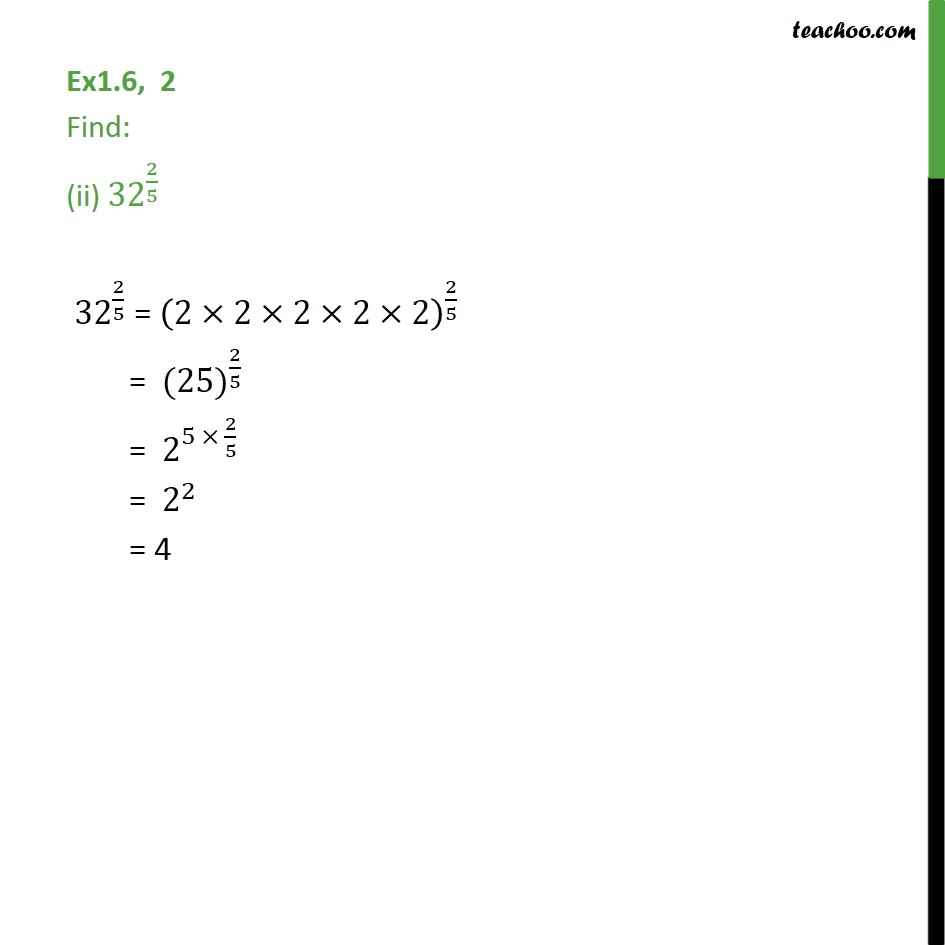1. Chapter 1 Class 9 Number Systems (Term 1)
2. Serial order wise
3. Ex 1.6

Transcript

Ex1.6, 2 Find: (ii) 〖32〗^(2/5) 〖32〗^(2/5) = (2×2×2×2×2)^(2/5) = 〖 (25)〗^(2/5) = 〖 2〗^(5 × 2/5) = 〖 2〗^2 = 4